Browse by Topic

# Wolfram|Alpha Pro Teaches Step-by-Step Arithmetic for All Grade Levels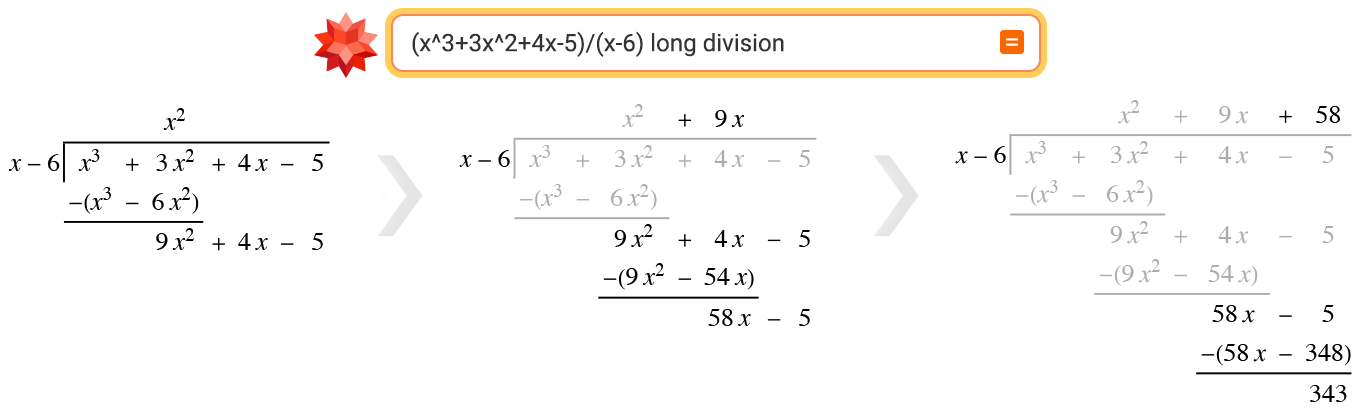In grade school, long arithmetic is considered a foundational math skill. In the past several decades in the United States, long arithmetic has traditionally been introduced between first and fifth grade, and remains crucial for students of all ages.

The Common Core State Standards for mathematics indicate that first-grade students should learn how to add “a two-digit number and a one-digit number.” By second grade, students “add and subtract within 1000” and, in particular, “relate the strategy to a written method.” In third grade, multiplication by powers of 10 is introduced, and by fourth grade students are tasked to “use place value understanding and properties of operations to perform multi-digit arithmetic,” including multiplication and division. A fifth grader will not only be expected to “fluently multiply multi-digit whole numbers using the standard algorithm,” but also “add, subtract, multiply, and divide decimals.”

Now, Wolfram|Alpha Pro returns step-by-step solutions for long addition, subtraction, multiplication and division problems, including ones involving decimals or negative numbers. We have also developed detailed step-by-step solutions for long division of whole numbers and negative numbers as well as—for the high-school level—multiplication and division of polynomials.

## Long Arithmetic in Wolfram|Alpha

Long arithmetic is used to solve addition, subtraction, multiplication and division problems in writing, often by organizing numbers one on top of the other, with digits aligned in columns.

The long arithmetic algorithms are rooted in the concept of place value. In our base-10 number system, each digit represents a count of a certain value associated with its place in the number. For example, a three-digit number with no decimal uses three place values: the hundreds, tens and ones. Aligning numbers based on their digits amounts to lining up the digits with the same place values. While the long arithmetic algorithms can be carried out without fully thinking through the place value reasoning each time, it can be conceptually useful for students to understand the process of, for example, multiplying the ones, multiplying the tens and multiplying the hundreds, then combining those results to get the final result.

Long arithmetic can be challenging for students seeing it for the first time. Moreover, since there is a variety of long arithmetic methods, it can be challenging for parents to help their students. If it seems to you like every generation learns a new long arithmetic method, that may not be your faulty memory! In fact, there are many variations of the long arithmetic algorithm, and which one you learn in school can depend on a variety of factors, from geographical region to teacher preference to curriculum updates.

## Step-by-Step Problem Solving✕

If you ask Wolfram|Alpha to add several numbers, you can view a step-by-step solution that performs the computation using long addition. First, we arrange the numbers into columns based on place value, using the decimal point as a guide: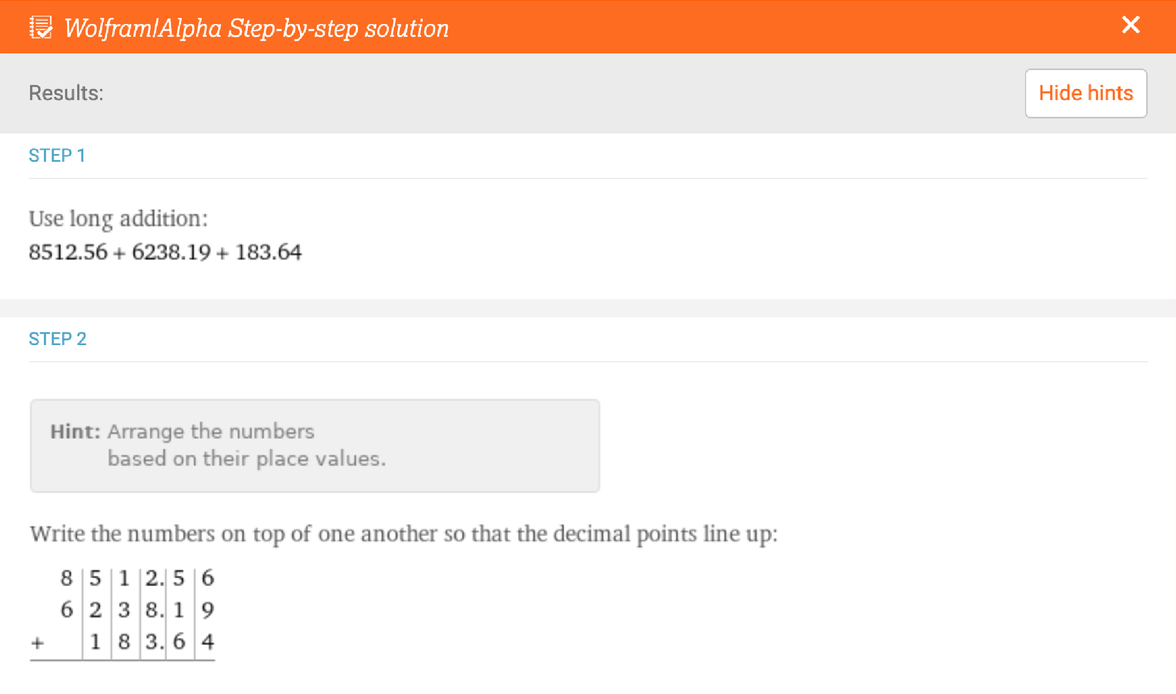✕

Wolfram|Alpha then walks you through each step of the long addition algorithm. In general, this involves adding the digits in each column from right to left. If the digits in a column sum to 10 or more, we carry the first digit to the column to the left.

More conceptually, adding the digits in a column means counting the number of units in a particular place value. In the following step, for example, summing the 6 + 9 + 4 in the hundredths column adds up to 19 units in the hundredths column. Nineteen hundredths is equivalent to 1 tenth and 9 hundredths, and so we record the 9 hundredths in the hundredths column and move the 1 tenth to its fellow tenth units in the tenths column: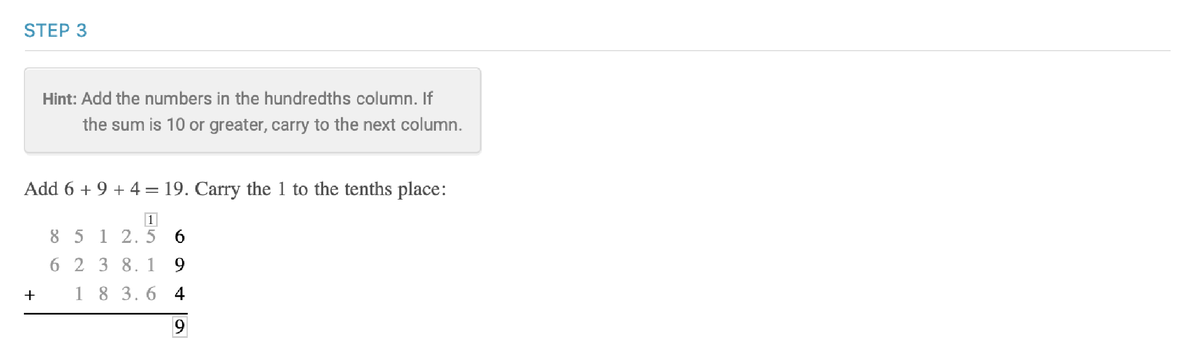✕

The step-by-step solution guides you through the addition of digits in each column, at which point you can read the final answer from the bottom of the grid: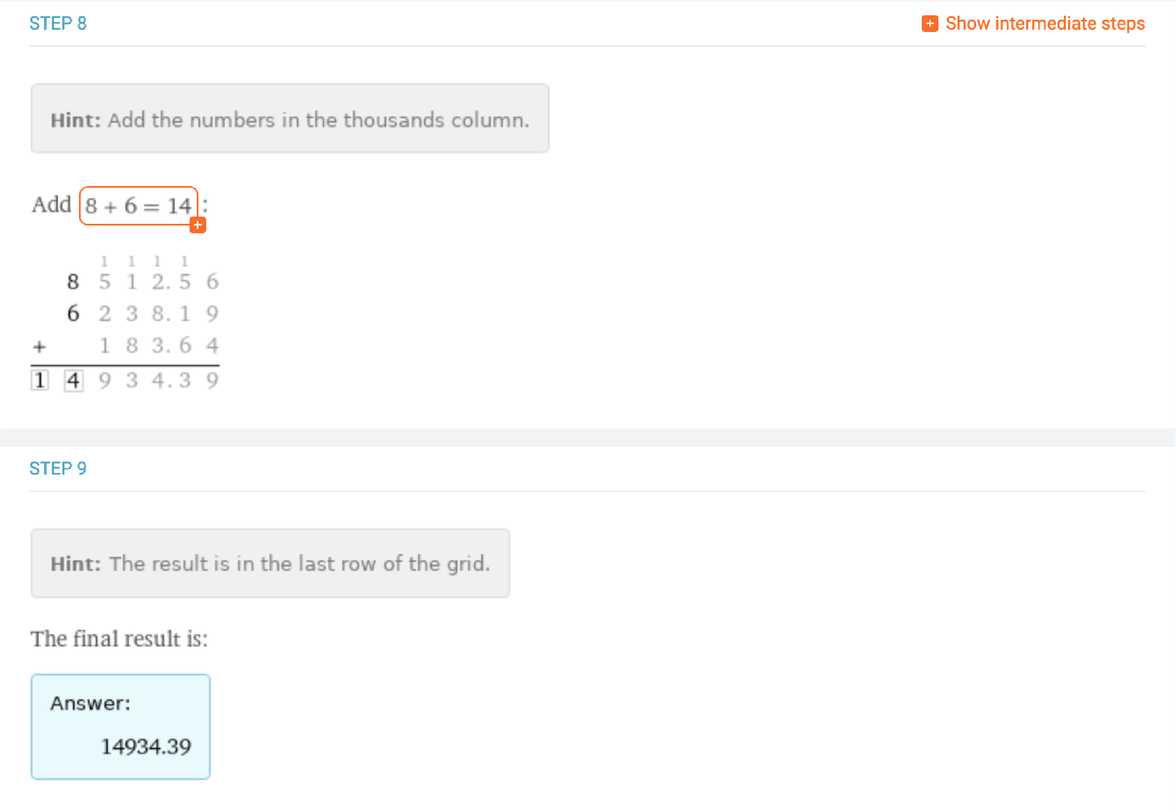✕

### Long Subtraction✕

Wolfram|Alpha also returns step-by-step solutions for long subtraction of a smaller number from a larger one. We begin setting up the problem by arranging the numbers on the page: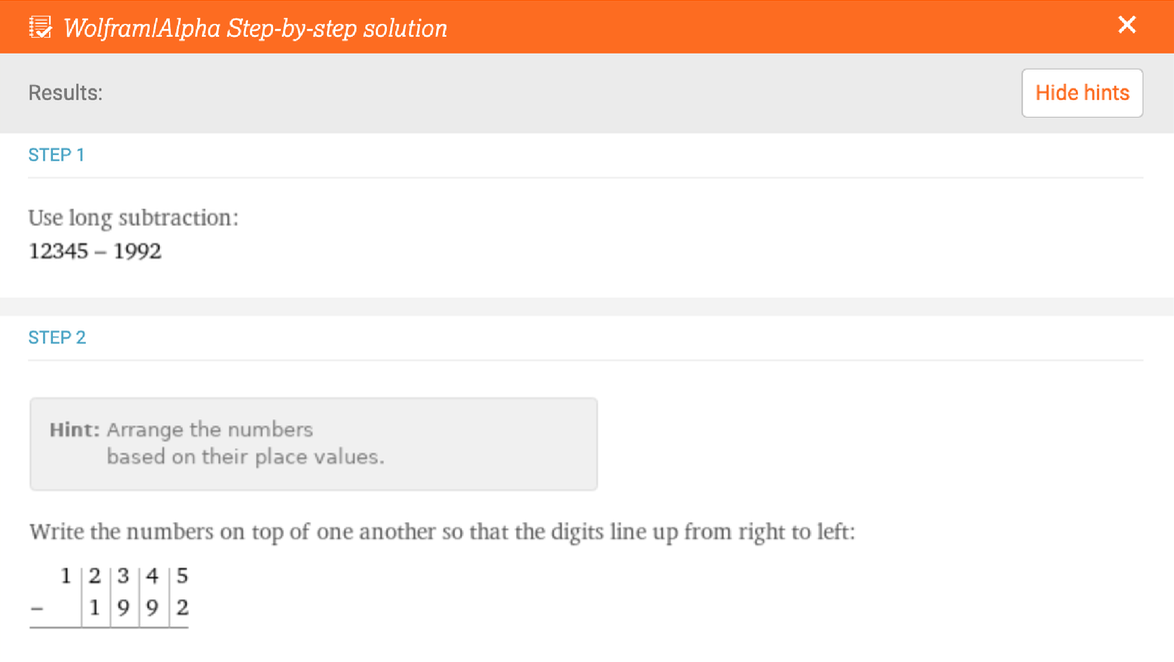✕

The long subtraction algorithm proceeds by subtracting the bottom digit from the upper digit in each column. In the case that the bottom digit is a higher number than the upper digit, we must borrow from the columns to the left. We indicate this on the long subtraction grid by replacing the 3 in the hundreds column with a 2 and moving the borrowed 1 into the tens column to create 14 tens: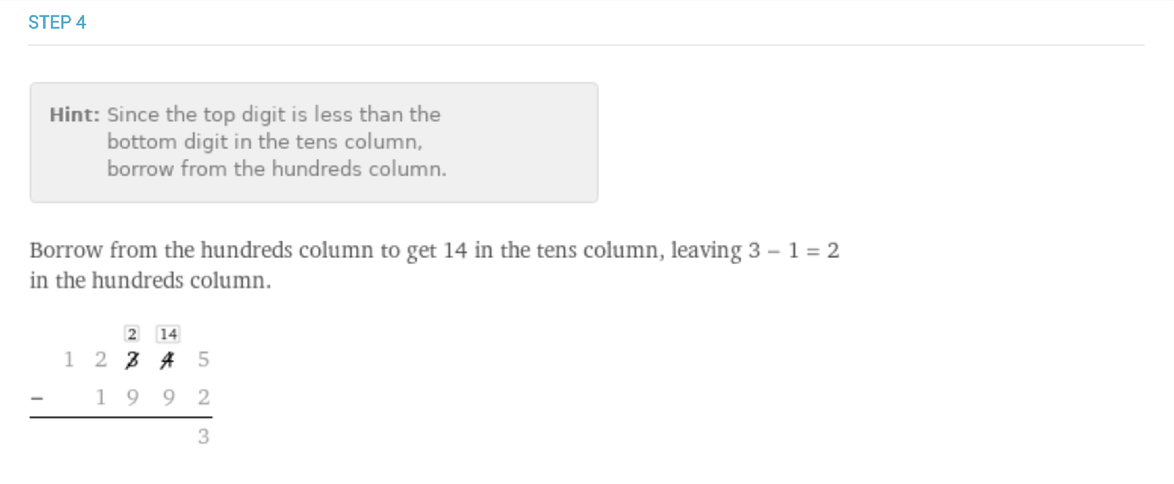✕

The long subtraction–borrowing procedure can be explained in terms of place values. In the previous step, the need to borrow arises in the tens column because 4 tens is fewer than 9 tens. We therefore look beyond the tens column to the hundreds column, which allows us to instead consider subtracting 9 tens from 1 hundred and 4 tens. Conceptually, this changes the relevant subtraction problem for this step from 40 – 90 to 140 – 90. In the long subtraction grid, this only appears as 14 – 9 = 5; the place values of the digits are encoded in their positions in the numbers in the long subtraction grid: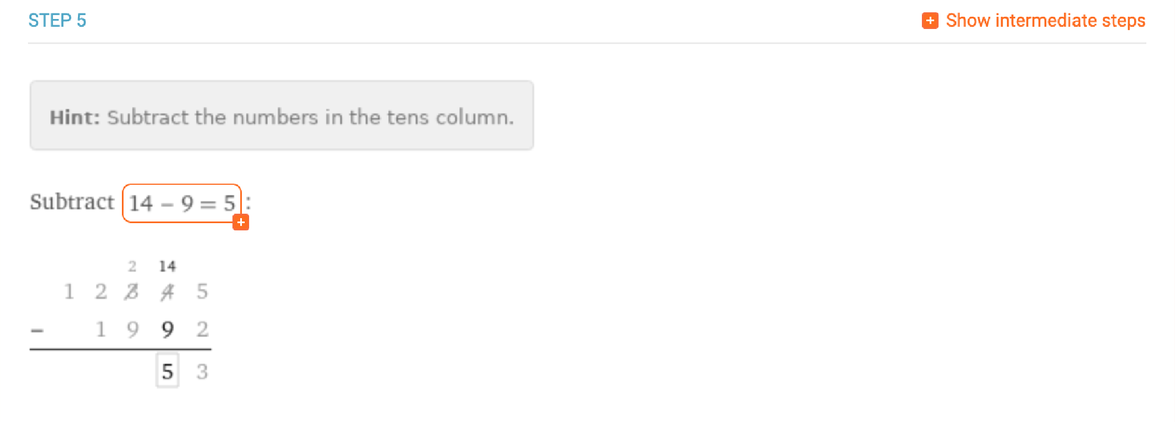✕

Each borrowing and subtracting step is enumerated in the step-by-step solution. When there are no longer any digits in the bottom number, we can bring down any remaining digits from the upper number and read off the final answer from the bottom of the long arithmetic grid: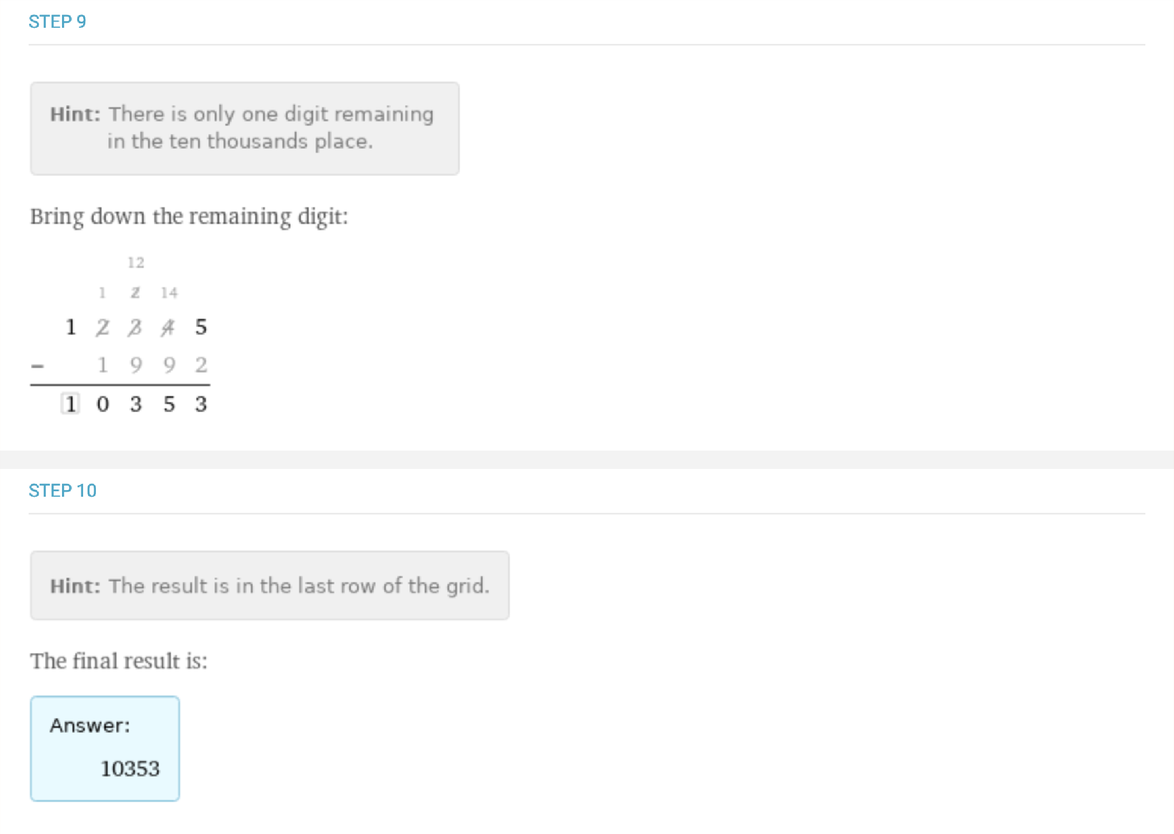✕

### Long Multiplication

For step-by-step long multiplication, we recently added the capability to multiply decimals and negative numbers. Performing the long multiplication algorithm with decimals or negative numbers simply involves replicating the algorithm as if for integers and then placing the decimal or negative in an additional step before reporting the final answer.

In the words of the great Jaime Escalante in the 1988 film Stand and Deliver, “A negative times a negative is a positive!” The step-by-step solution for multiplying two negative numbers explains that you can effectively ignore the negative signs before continuing with the long multiplication algorithm:✕

One step of the long multiplication algorithm involves multiplying a digit of the second number by each digit of the first, carrying the tens digit of each product as necessary. Each step is summarized in the step-by-step solution: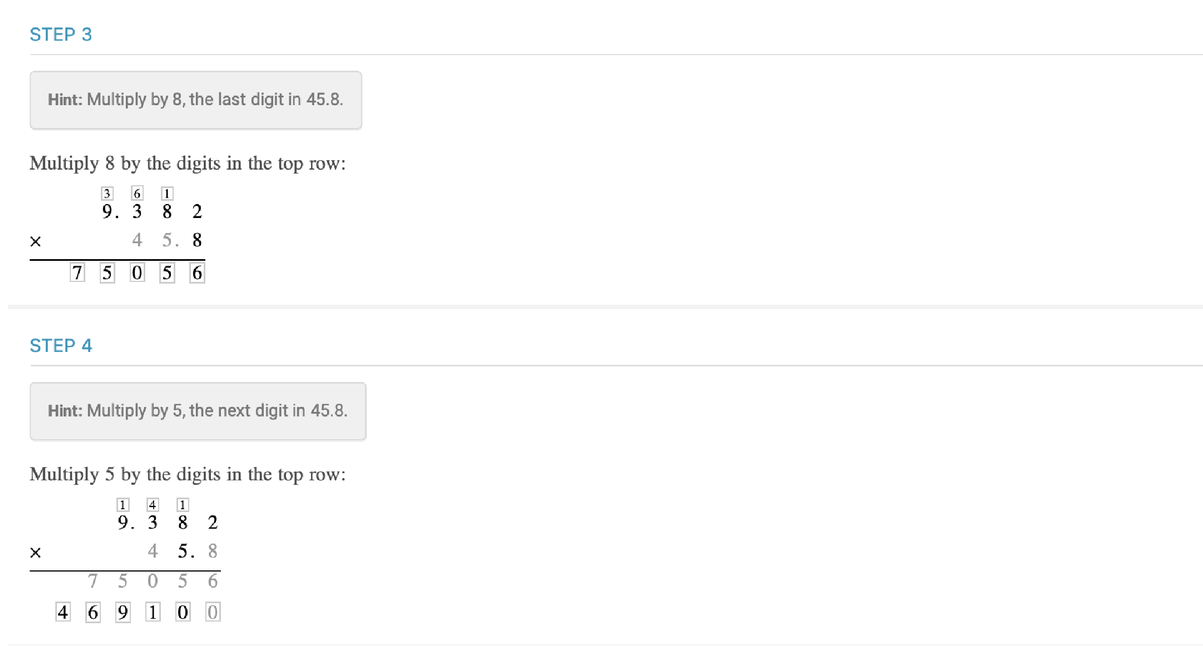✕

Finally, if we are multiplying decimal numbers, we write the decimal in the final answer by taking place value into account, which amounts to counting up the number of digits after the decimals in the original numbers: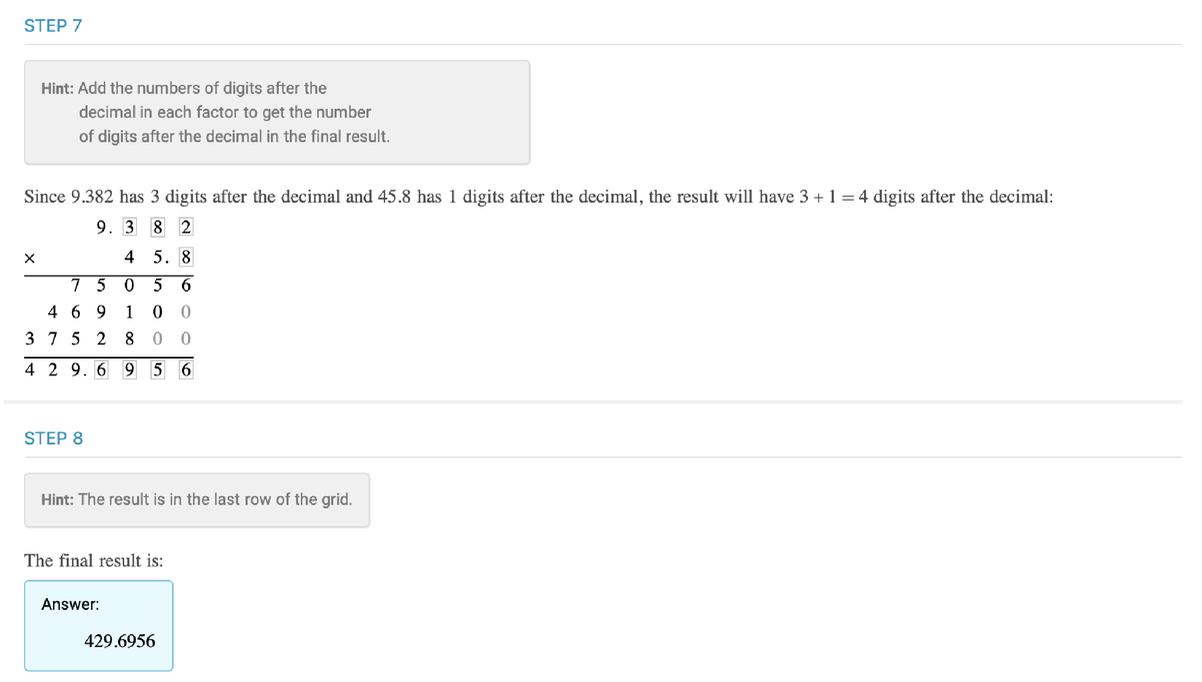✕

### Long Division

The final result of long division is not always given as a single number. When a number does not evenly divide into another, long division reveals both the quotient and remainder:✕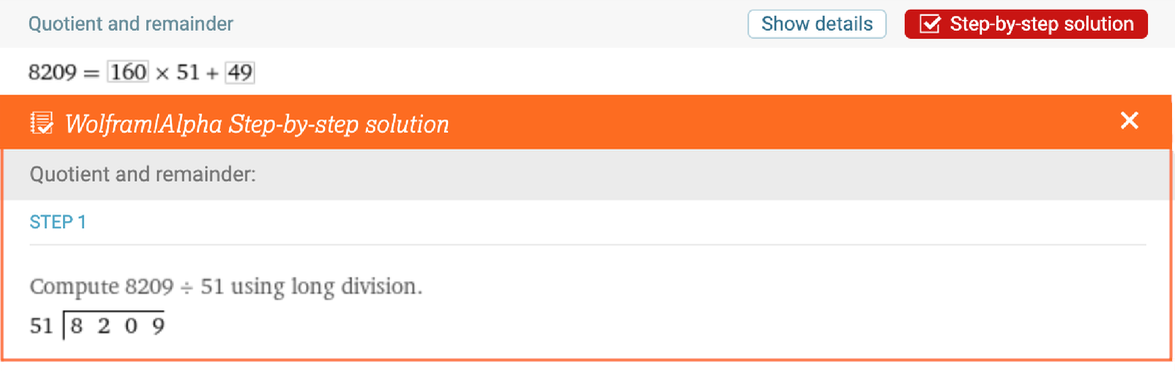✕

Another way to report the result of a long division problem is with a mixed number, sometimes referred to as a mixed fraction: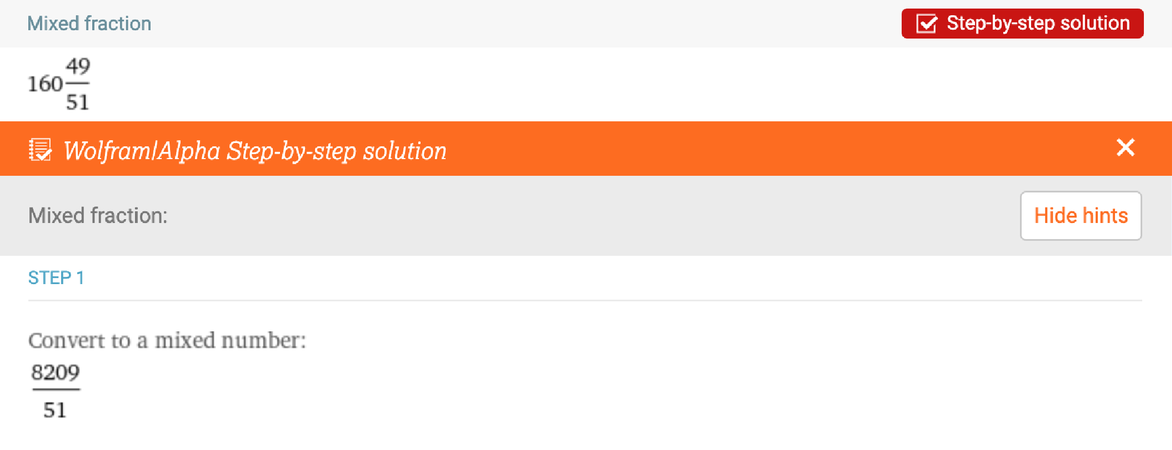✕

Regardless of how you are presenting the final answer, the steps for performing the long arithmetic algorithm are the same. We begin by arranging the numbers in a slightly different layout, using a division bracket instead of stacking the numbers vertically, as we did for the previous algorithms. The number to the left of the bracket is the divisor and the number inside the bracket is the dividend: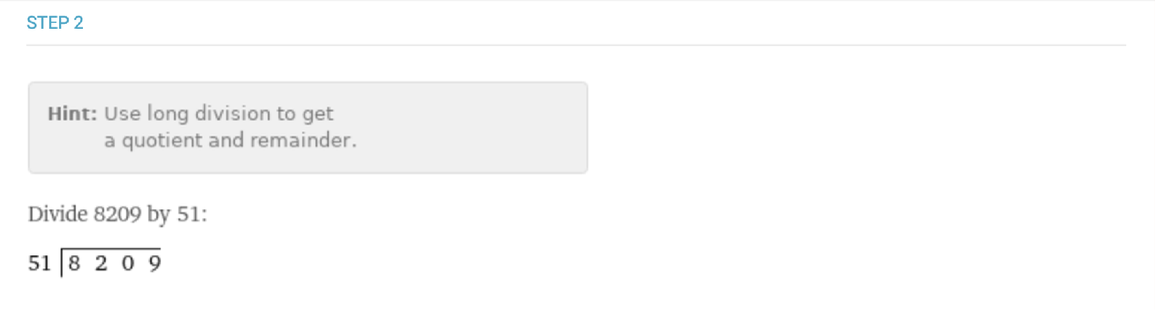✕

Each step of the long division algorithm requires multiple substeps. First, if the divisor has two digits, as in our example, you need to determine how many times the divisor goes into the first two digits of the dividend. Write that number on the top of the division bracket, multiply the divisor by that number, subtract that product from the divisor and bring down the next digit: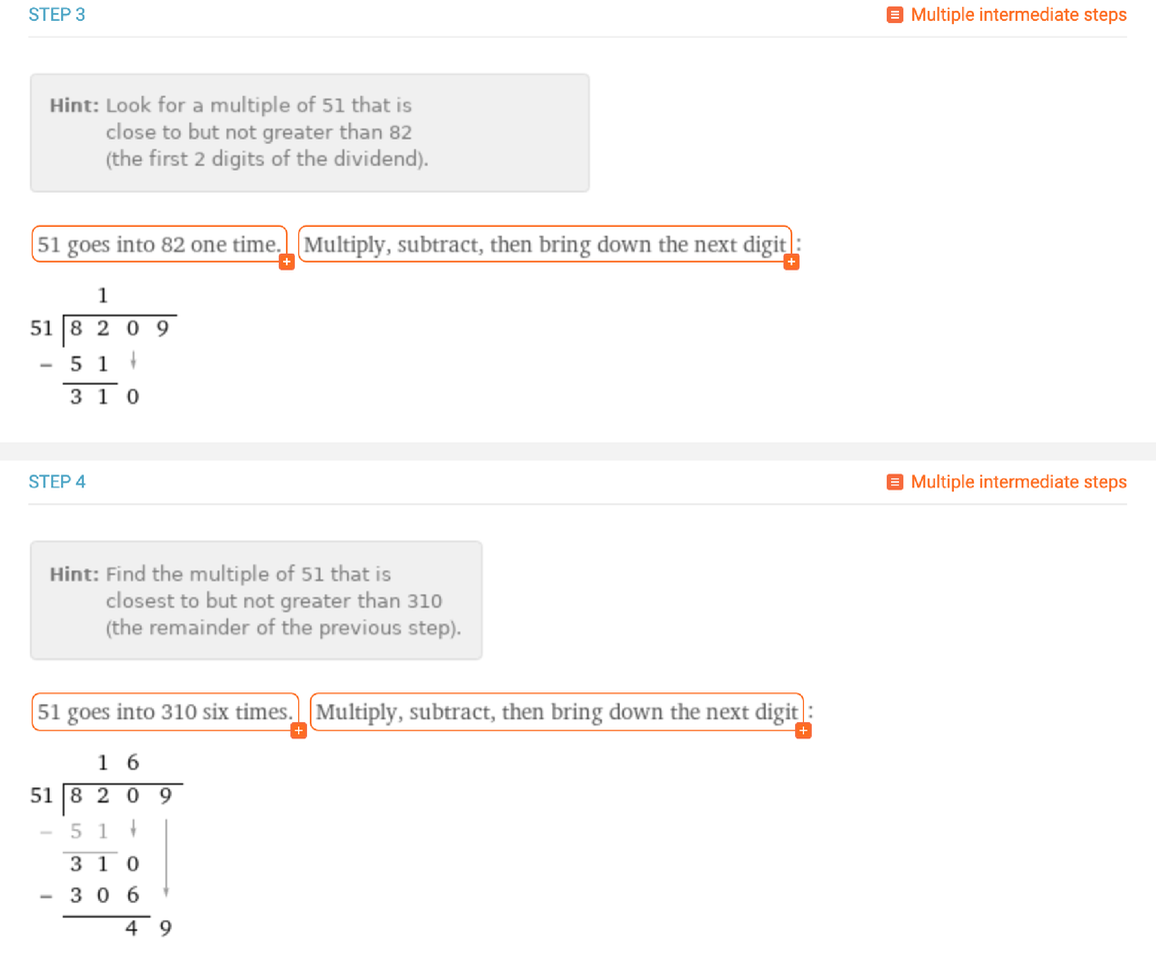✕

Phew! Need some extra clarification on that? If so, you’re not alone. Long division is a notoriously challenging long arithmetic method to learn or teach. Behind the Multiple intermediate steps button, therefore, you can see the multiplication and subtraction worked out separately, with each addition to the bracket explained one at a time: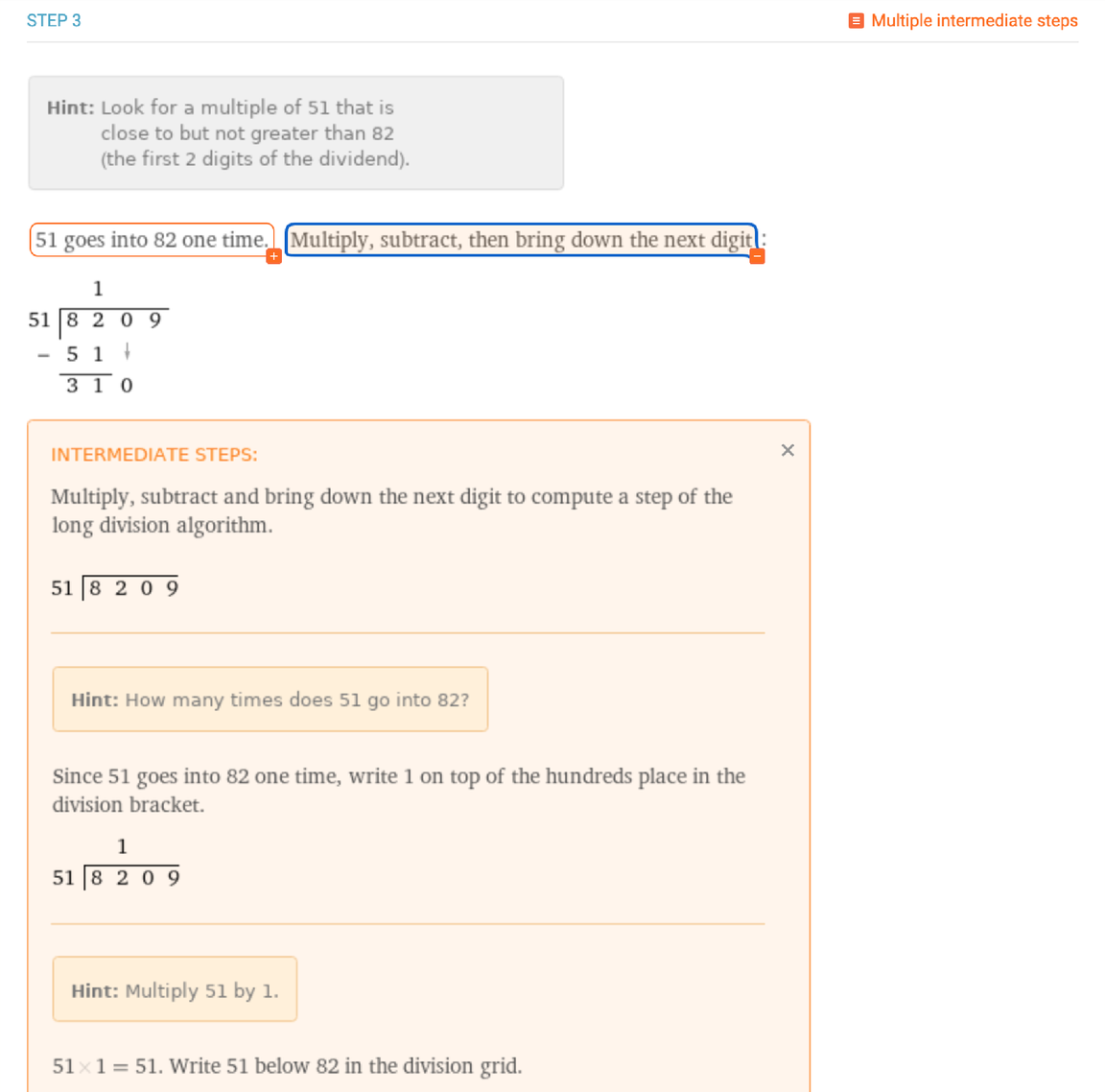✕

After performing the steps for the long division algorithm outlined until no more digits of the dividend remain, a final step guides you through the process for finding the quotient and remainder and, if desired, expressing the result as a mixed number: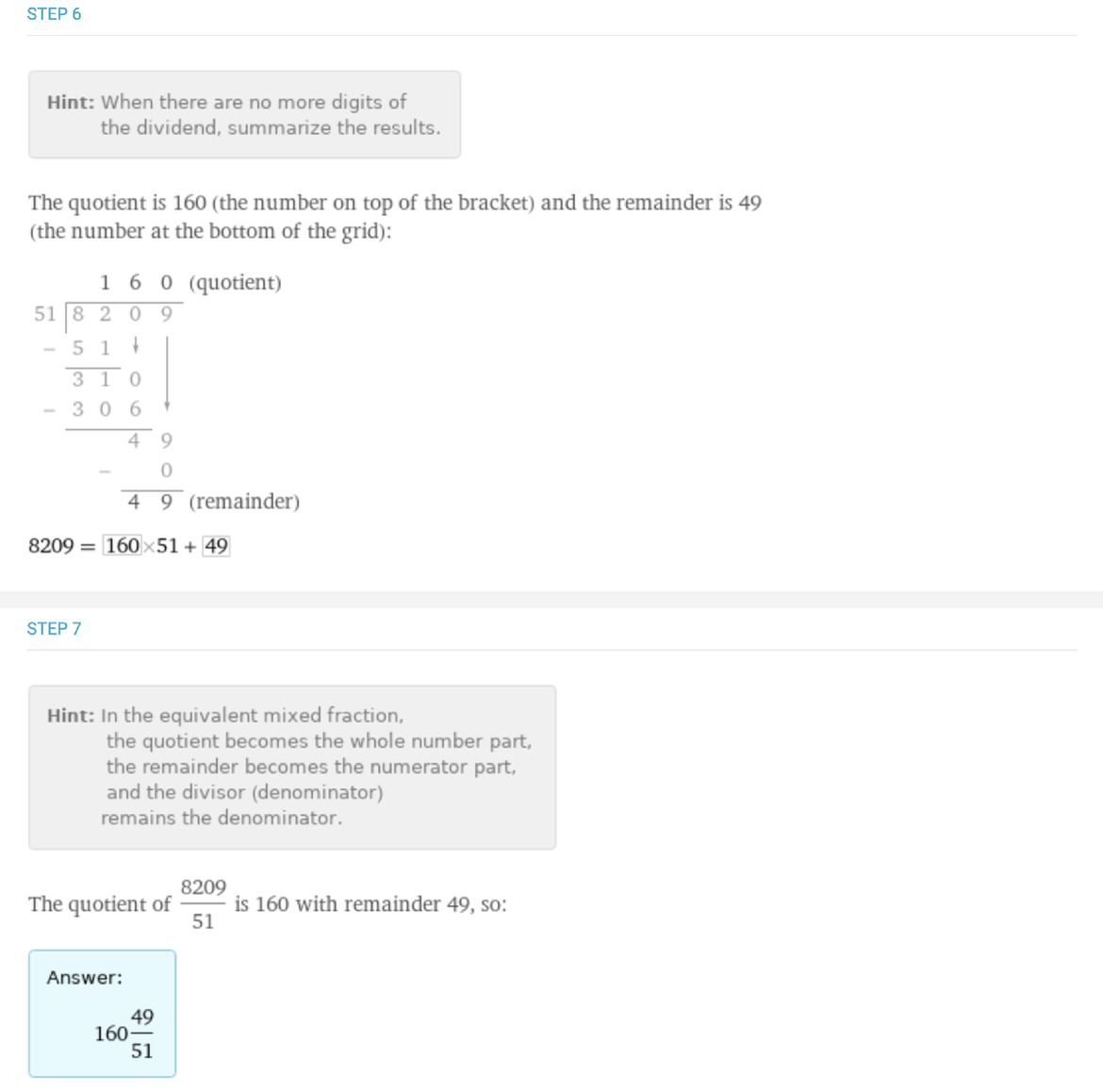✕

Presently, Wolfram|Alpha only returns step-by-step long division solutions for integers, not for decimal numbers. We look forward to expanding our step-by-step support for long division to include decimals in the near future.

## Polynomial Arithmetic

In high school, students extend long arithmetic with numbers to apply to mathematical expressions called polynomials. Polynomials are sums of terms that include variables and exponents, such as 3x2 + 4x – 5. Polynomials can be added, subtracted, multiplied and divided using methods analogous to numeric arithmetic. In particular, polynomial multiplication and division are critical skills for upper-level high-school and college math classes. We have recently expanded the step-by-step solutions for polynomial multiplication and division problems.

There are similarities between long arithmetic for numbers and for polynomials. While the digits in numbers can be grouped based on their place values, the terms in a polynomial can be grouped based on the exponents of the variable.

In high school, students learn how to “add, subtract, and multiply polynomials” and, as stated separately in a different Common Core Standard, divide polynomials “using inspection, long division, or, for the more complicated examples, a computer algebra system.” Wolfram|Alpha is the perfect tool for handling those complicated examples, especially because we also show how to arrive at the solution via step-by-step polynomial long division.

### “Box Method” Polynomial Multiplication

One common method for multiplying polynomials, often referred to as the “box method,” involves organizing the terms of the polynomials around the outside of a grid. The step-by-step solution explains how to determine the size of the grid: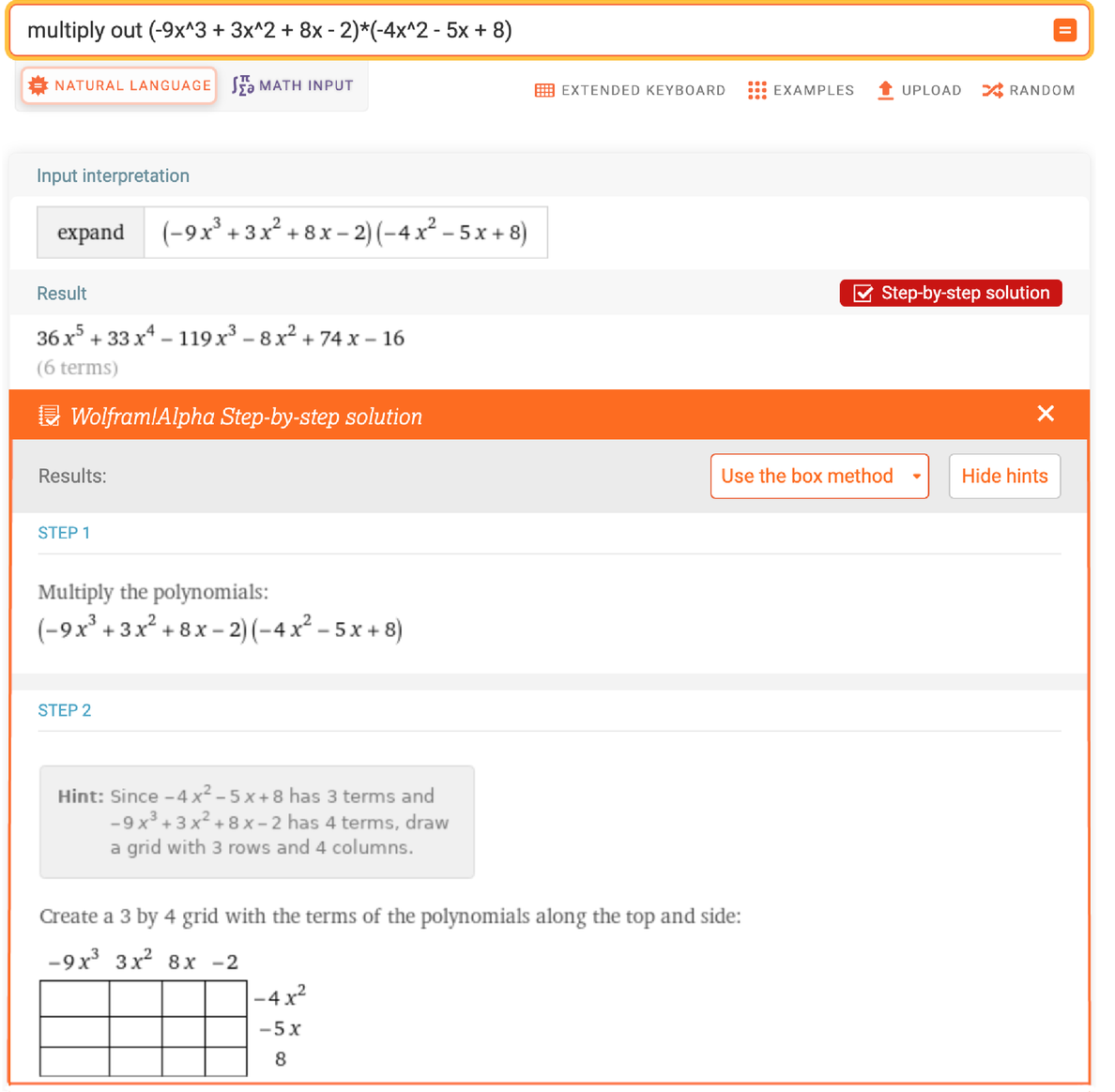✕

Next, we fill in each box in the grid by multiplying the terms in each row-column pair. Each of these steps is outlined in the step-by-step solution, and the intermediate steps give details about how to multiply each pair of polynomial terms: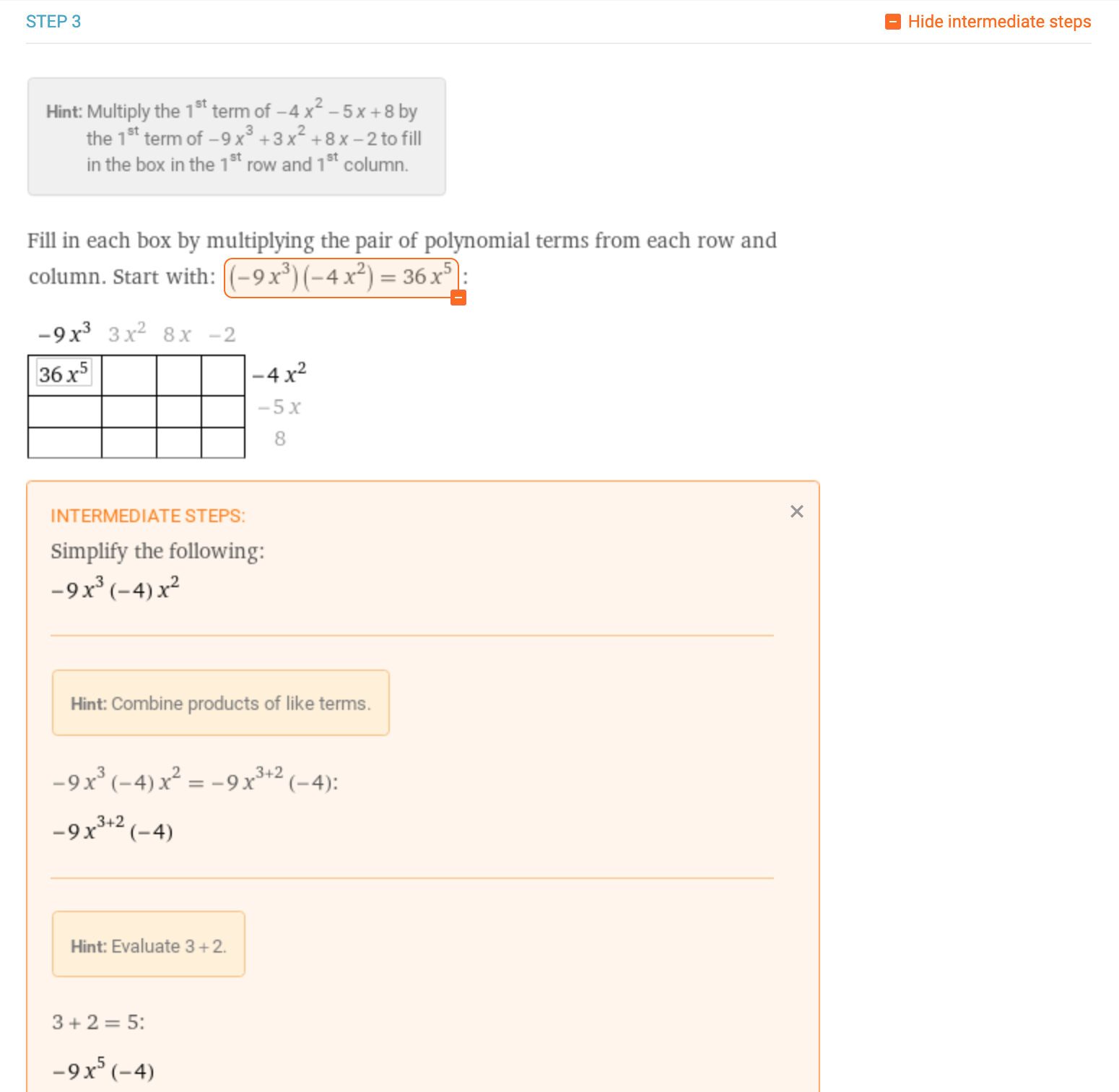✕

Finally, we obtain the result of the polynomial multiplication problem by summing the terms in all of the boxes: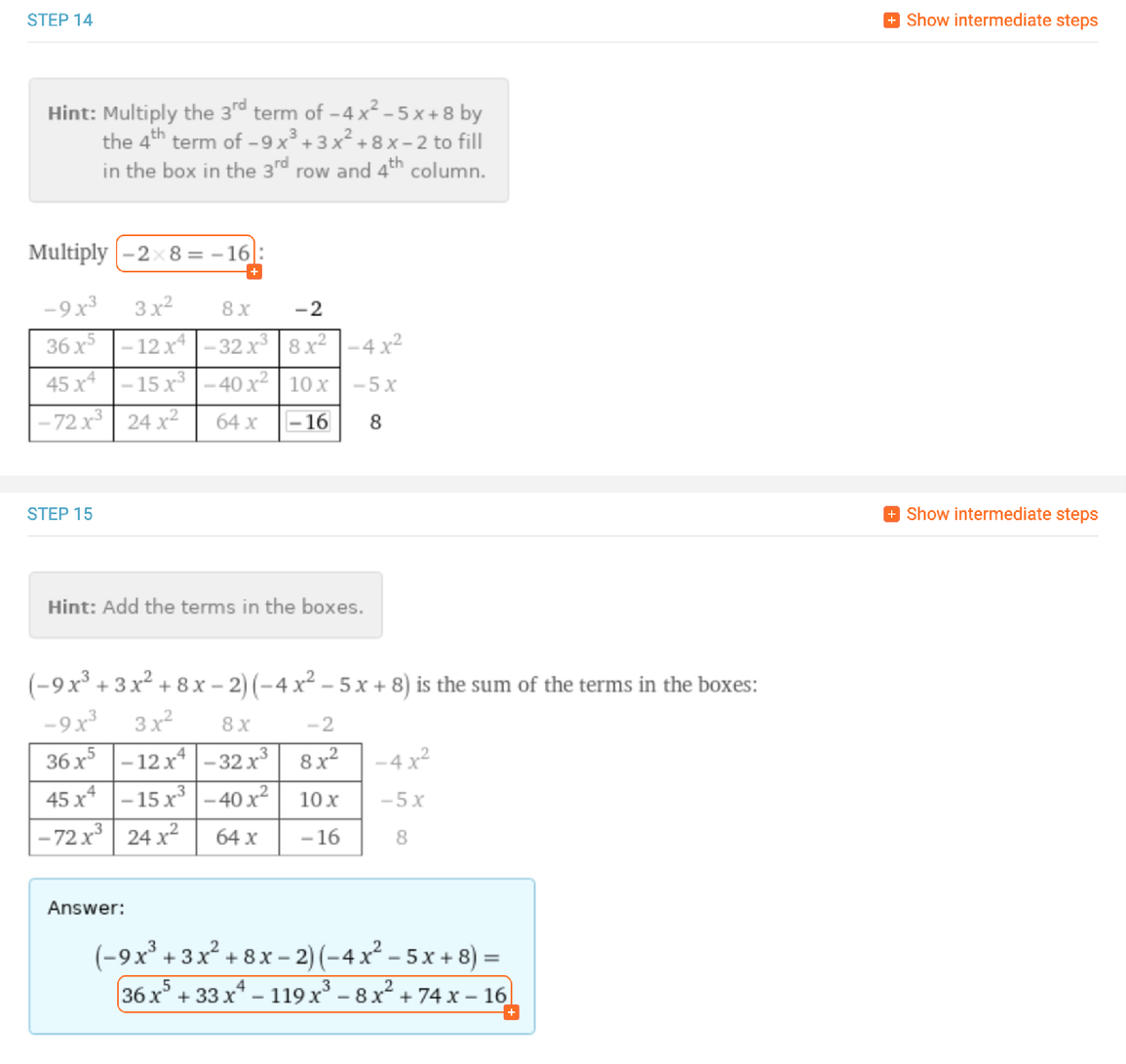✕

The box method is particularly useful as a visualization of the pairwise multiplication of the terms of each polynomial. We are also working on developing additional methods for polynomial multiplication, including polynomial long multiplication (formatted similarly to numeric multiplication) and the “arrow” and “FOIL” methods commonly taught in high schools in the United States.

### Polynomial Long Division

Last but not least, we also have improved step-by-step support for polynomial long division problems. Polynomial long division follows an algorithm similar to integer long division, but with extra requirements for keeping track of variables and exponents. To divide one polynomial by another, we set up the long division bracket with the dividend (or numerator) inside and the divisor (or denominator) outside: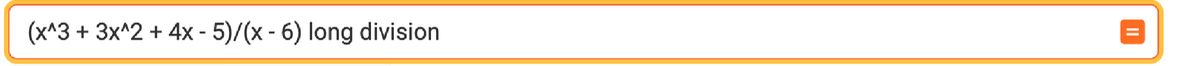✕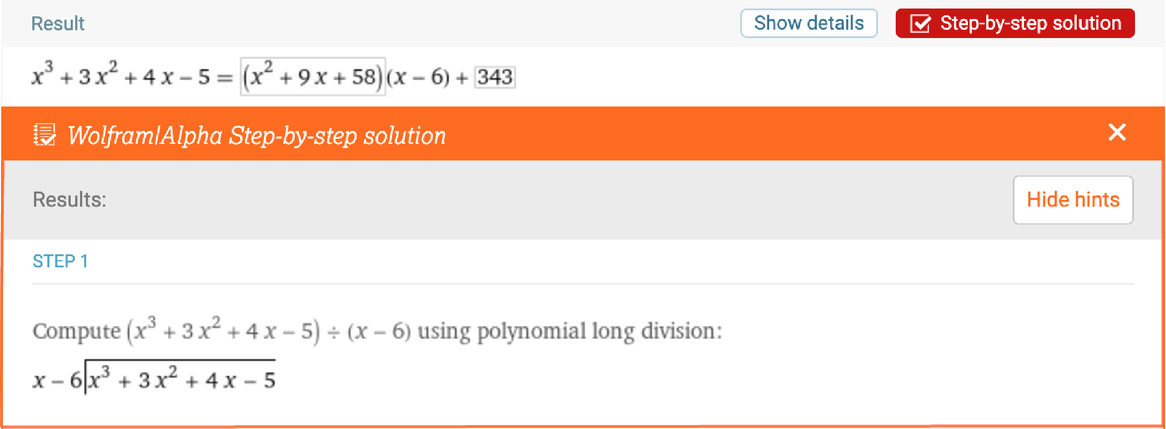✕

The “multiply, then subtract” structure of the integer long division algorithm is the same for polynomial long division. The question of “How many times does the divisor go into the first term(s) of the dividend?” can roughly be translated to something like “How many fewer highest powers of x does the divisor have than the first term of the dividend?” Mathematically speaking, we refer to the “leading term” as the term of the dividend with the highest power of x and determine what the divisor needs to be multiplied by to match the leading term of the dividend. The multiple is recorded on top of the bracket, and the result of the multiplication is recorded and subtracted at the bottom of the grid. Since students doing polynomial long division are already familiar with integer long division, this entire step is summarized inline: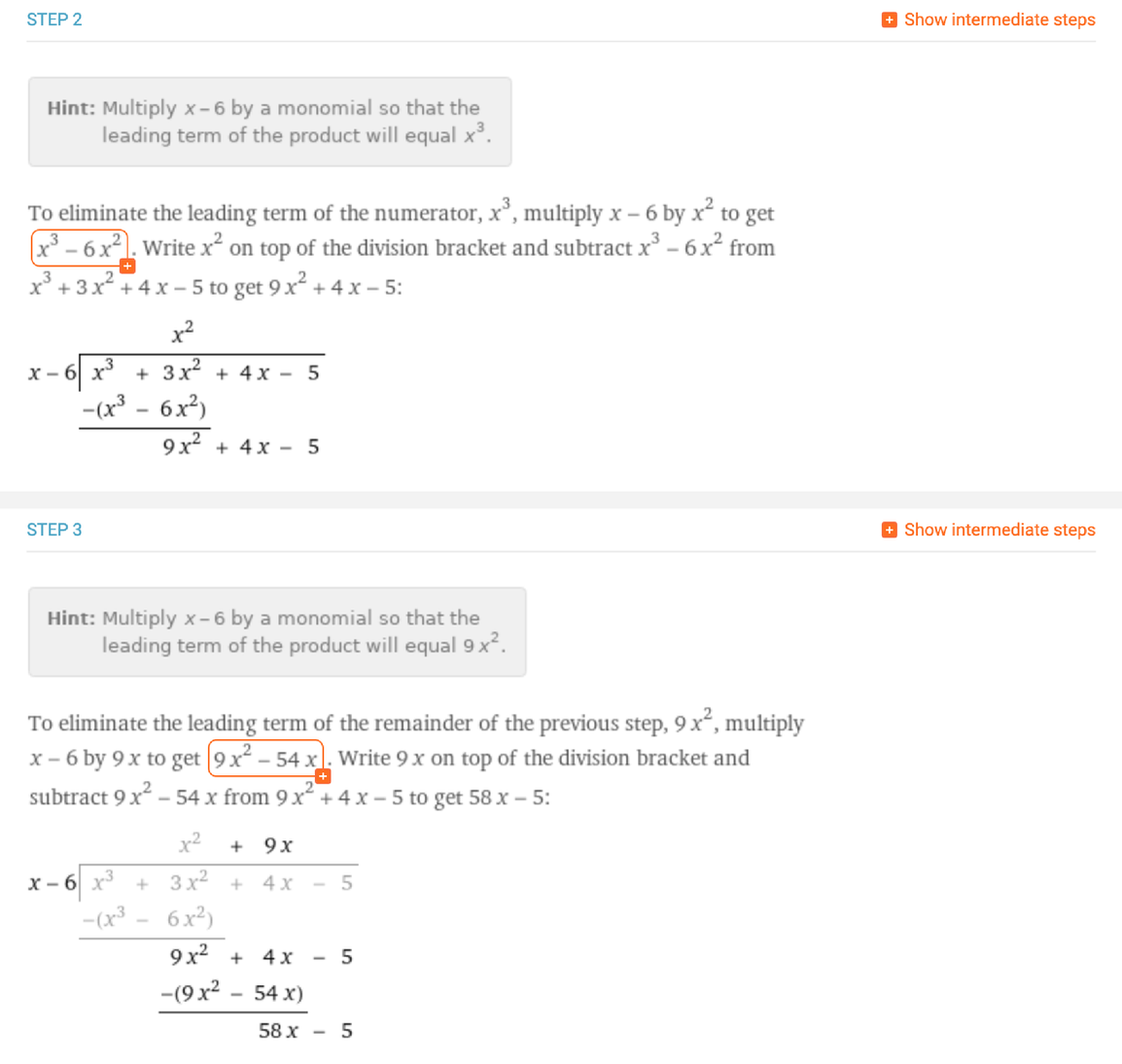✕

Once we have completed the final subtraction, the quotient and remainder appear in the same places of the long division grid as they do for integer long division. The final result can be reported either in terms of a fraction or in terms of multiplication of the quotient by the divisor, in what we refer to as “quotient and remainder form”: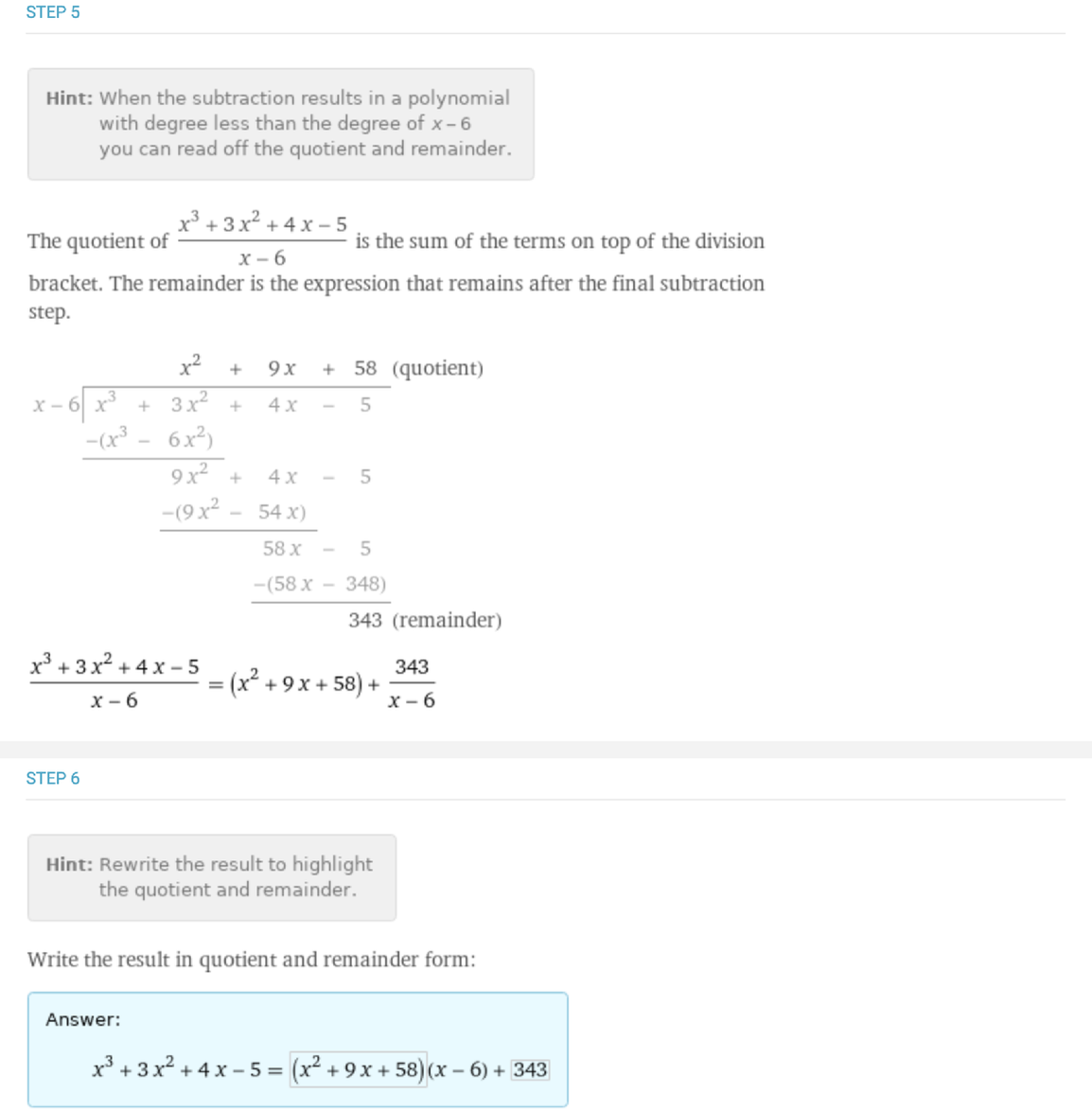✕

## Future Work

The Wolfram|Alpha math team hopes to further expand its step-by-step coverage for long arithmetic. We continue to work on adding more methods for long arithmetic, including alternate representations of the same algorithms. While the underlying conceptual arithmetic algorithms do not vary among teachers or regions, there is some variation in the procedures and methods for representing long arithmetic problems. Different teachers have different preferences of methods for communicating content to their students, and the arrangement of columns and procedures for visualizing arithmetic vary regionally.

We are also working to add more methods beyond the traditional long arithmetic algorithms, such as visual methods that emphasize different mathematical concepts behind the arithmetic. We hope to provide useful tools for students, parents and teachers performing long arithmetic, and welcome feedback or requests if there are particular additions (or subtractions, multiplications and divisions) you would find useful.

 Sign up for Wolfram|Alpha Pro to access customizable settings, step-by-step solutions, increased computation time and more.